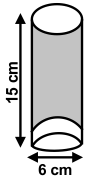# CREST Mathematics Olympiad Class 10 Previous Year Papers

## Syllabus:

Section 1: Real Numbers, Polynomials, Pair of Linear Equations in Two Variables, Quadratic Equations, Arithmetic Progressions, Triangles, Coordinate Geometry, Introduction to Trigonometry, Some Applications of Trigonometry, Circles, Constructions, Areas Related to Circles, Surface Areas and Volumes, Statistics, Probability.

Achievers Section: Higher Order Thinking Questions - Syllabus as per Section 1

 Q.1 In the adjoining figure, the bottom of the glass has a hemispherical raised portion. If the glass is filled with orange juice, then find the quantity of juice which a person will get:Q.2 If p1 and p2 are two odd prime numbers such that p1 > p2, then p12 - p22 is:
 Q.3 30% of the items were sold at a profit of 40% while the remaining were sold at x% loss. If the overall loss is 10%, find the value of x:
 Q.4 In the binomial expansion of (a - b)n, n ≥ 5 the sum of the 5th and 6th terms is zero. Find the value of a/b:
 Q.5 The average marks obtained by the students in a class are 43. If the average marks obtained by 25 boys are 40 and the average marks obtained by the girl students are 48, then what is the number of girl students in the class?
 Q.6 Perpendiculars are drawn from the vertex of the obtuse angles of a rhombus to its sides. The length of each perpendicular is equal to a units. The distance between their feet being equal to b units. Find the area of the rhombus:
 Q.7 If the sum of the roots of the equation ax2 + bx + c = 0 is equal to the sum of their squares, then which one of the following is correct?
 Q.8 Find the quadratic equation whose roots are reciprocal of the roots of the equation 3x2 - 20x + 17 = 0:
 Q.9 What is the value of the expression [(a - b)3 + (b - c)3 + (c - a)3] / [(a - b)(b - c)(c-a)]?
 Q.10 For an acute angle θ, sin θ + cos θ takes the greatest value when θ is:

Answers to Previous Year Questions from CREST Olympiads:

 Q.1 b Q.2 a Q.3 c Q.4 b Q.5 a Q.6 c Q.7 c Q.8 a Q.9 d Q.10 b

Previous Year PDF of CREST Mathematics Olympiad for Class 10: